Follow

## Default Weighting Behavior

By default, all columns are weighted based on the number of points designated to the column. So for example, you may have a course that’s evaluated based on 250 total points. You can then divide the 250 points into individual assignments, where the sum of the columns totals 250. So here’s an example: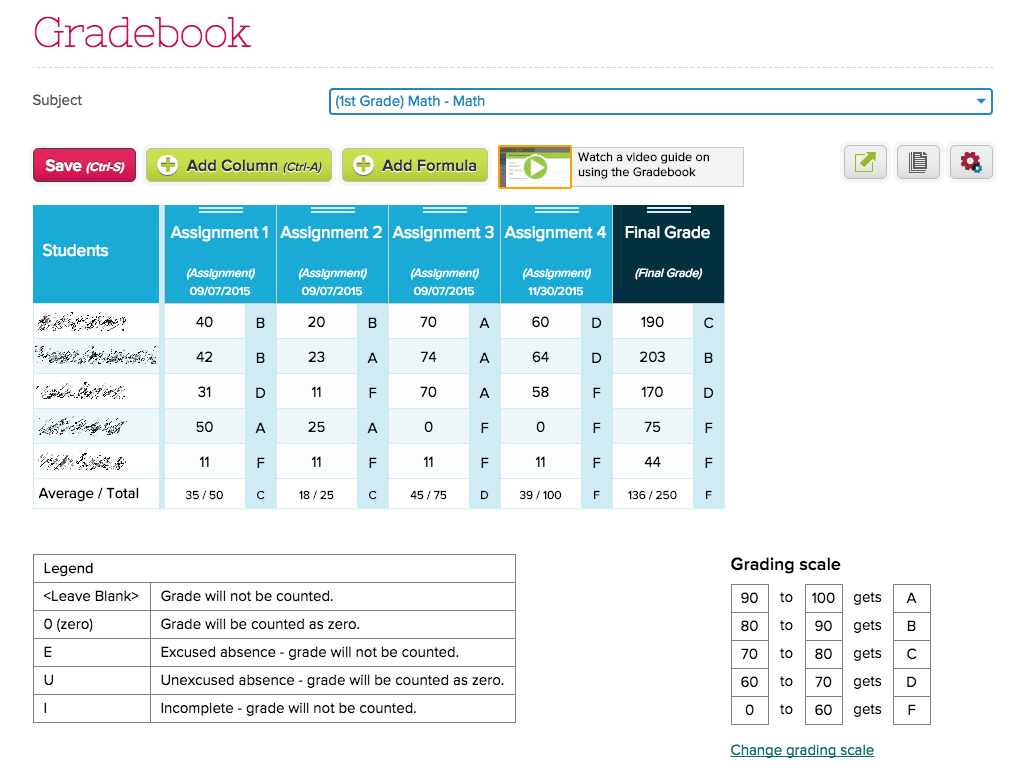In this example, Assignment 1 is worth 50 points, while Assignment 2 is worth 25 points. This means that Assignment 1 is worth twice that of Assignment 2. And in fact, the final score is simply the sum of all the points scores for each column. But if you want to display the final score as a percentage, just click on the “250” at the bottom of the column, and change it to “100”. That will renormalize the calculation: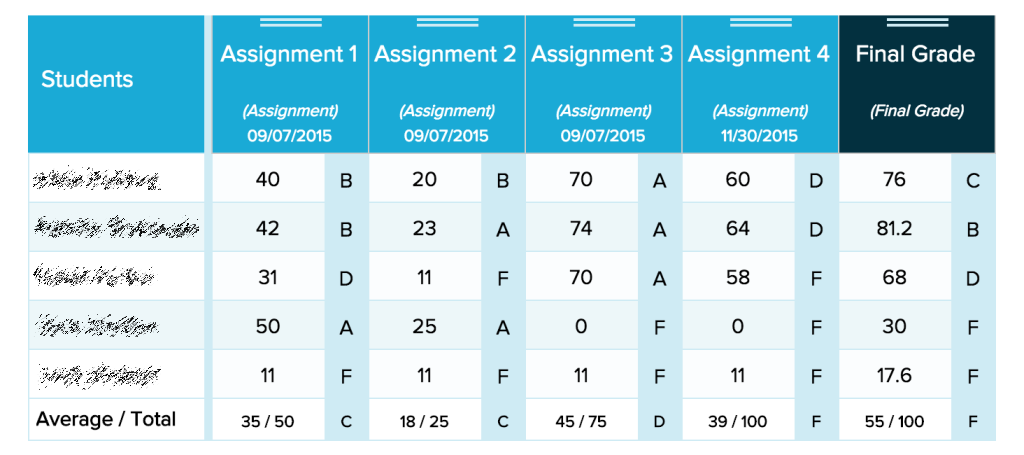## Weighting by Category

The first example above assumes that you’re not weighting the columns based on categories. To do that, you can click on the “(Final Grade)” link, and change the weightages:

Notice that you can select multiple categories for each weight factor. And if you need more categories, you’ll need to speak to your administrator to either (1) add the new categories, or (2) give you access to add your own categories.

Each weight factor will be converted into the percentage score using the default weighting behaviour discussed above.

You can actually have multiple formulas in your Gradebook. Some scenarios that may call for multiple formulas:

• You want to calculate and store a mid-term grade
• You want to calculate a portion of your grade, say for “Formative” vs “Summative”.

Regardless of the situation, note that you can only designate ONE formula as the final grade. This is the grade that will be transferred to the Report Cards module when the marking period is active. Once the report cards are locked, you’re free to change the formulas again, and perhaps designate a different formula as the final grade for the next marking period. Just note that if you do happen to select multiple formulas as “is final grade”, the actual grade that gets transferred to the report cards may be unpredictable.

## Weighting Individual Columns

There are some situations where you want to modify how much an assignment is worth, without changing the total number of points for that column. So let’s say from our original example, the total score is over 250 points, but even though Assignment 2 is worth 25 points, the assignment only has 10 questions. And so you want to scale the 10-point assignment to 25 points: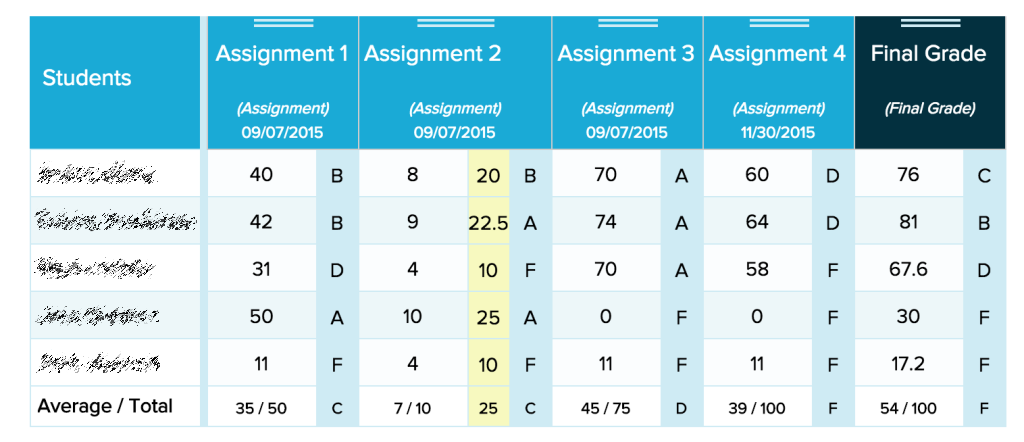To make this change, you can click on the Gears icon, and change the “Scale score total” to “25”, while leaving the “Total Marks” as “10”:

You can check the box labelled “Show scale score column” to view what the scaled value looks like on your Gradebook.

## Applying the same weight to All Columns

If for some reason you want to weigh all columns equally, you can use the “Scale score total” feature, and make sure it’s the same for all columns. Here’s an example where all columns are scaled to 100 points: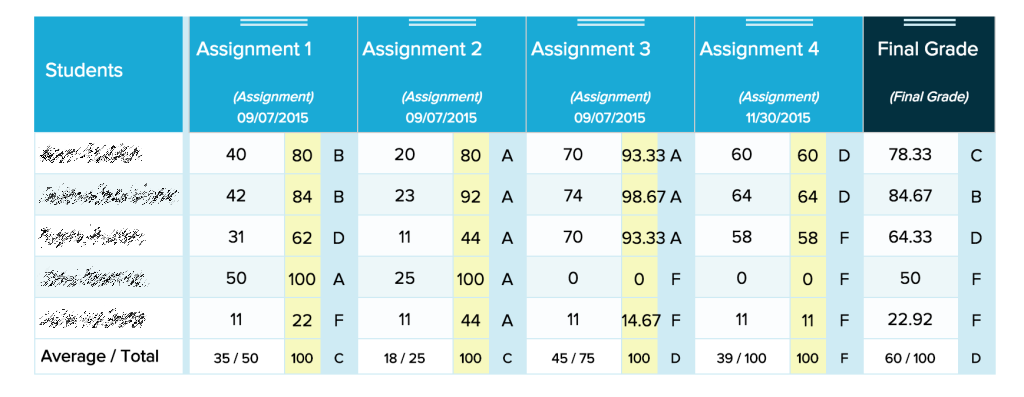Notice that calculated final scores are different. It’s now simply an equal average of all columns.

## Point Floor (Equal Interval)

This feature is primarily for a version of the Equal Interval scale, where if a student scores below a point floor, the student automatically receives the minimum score for that column. There are 2 areas where this can happen:

### Gradebook Column - “Scale Score minimum percentage”

When editing a Gradebook Column, you can specify the “Scale score minimum percentage” for that column:

Here’s what the Gradebook will look like if the 50% minimum is applied to all columns: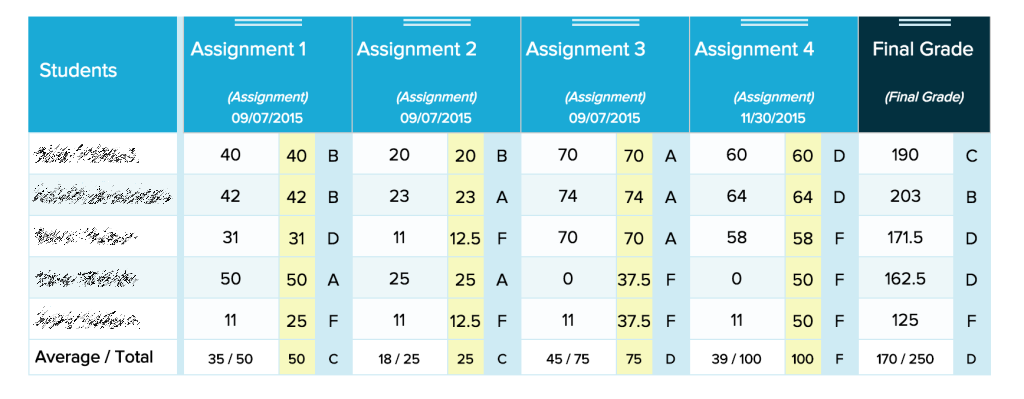Gradebook with Scale Score Minimum Percentage

Notice that when a student scores below 50%, the actual score being using is 50% of the assignment score.

### Grading Scale - “Minimum percentage (optional)”

You can use this to set the minimum score when you use a Legend where a zero is applied. So for example, a “U” which stands for “Unexcused Absence” would typically be worth zero points. But when you use a Grading Scale where the Minimum Percentage has been set, the student will receive the minimum percentage set by the grading scale.

IMPORTANT NOTE: If you’re using the Point Floor feature in your gradebook, you’ll need to use BOTH methods, as they are complementary to one another. The first method is for completed assignments, while the second method is for when a legend is applied.

With these additional features for calculating final averages, teachers have much more flexibility to customize their grading formula. If you would like this enabled for your school, or have any questions on this or how to setup your calculations, please do feel free to contact us any time!